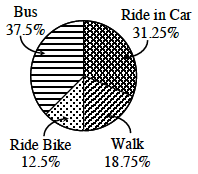### Home > CC3 > Chapter 7 > Lesson 7.1.1 > Problem7-8

7-8.

A group of classmates surveyed how other students travel to school. The results of the survey are shown in the table below. Make a circle graph showing the results of the survey using percents.  Homework Help ✎

 Mode of Transportation Number of Students Bus $90$ Ride Bike $30$ Ride in Car $75$ Walk $45$

Find the students using each mode of transportation as a percentage of all the students.

Each category of data is then represented by a portion of the circle. The measure of the angle of each sector portion is directly proportional to the corresponding percentage.

Consider the bike riders.

$\frac{30}{240}=0.125$ of the student population ride bikes to school.

Proportionately as an angle, this would be $12.5\%$ of$360°$ (a whole circle). This equates to $0.125(360°)=45°$.